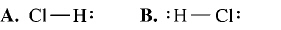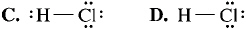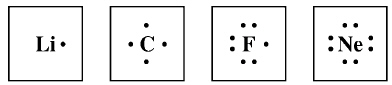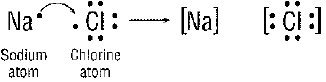﻿ Bonding Quiz
 Name:    Bonding Quiz

Modified True/False
Indicate whether the statement is true or false.  If false, change the identified word or phrase to make the statement true.

1.

The compound with the formula K2O is called potassium oxygen.

2.

When an atom gains an electron it becomes a positive ion.

3.

When electrons are transferred between two atoms, a covalent bond is formed.

4.

The attraction between a positive ion and a negative ion results in a covalent bond.

5.

Orderly crystal shapes, high melting points, and electrical conductivity when dissolved in water are properties of ionic compounds.

6.

Molecules are neutral.

7.

Low melting points and lack of electrical conductivity are properties of molecular compounds.

8.

Because a water molecule has a slight positive charge at one end and a slight negative charge at the other end, it is a nonpolar molecule.

Multiple Choice
Identify the choice that best completes the statement or answers the question.

9.

Which of the following compounds is covalent compound?
 a. H2O b. MgCl2 c. Na2S d. CaF2

10.

In the three molecules, O2, HCl, and F2, what atom would have a partial negative charge?
 a. fluorine b. chlorine c. oxygen d. hydrogen

11.

What kind of chemical bond is formed when a transfer of electrons occurs?
 a. ionic b. covalent c. magnetic d. hydrate

12.

What is the correct formula for the compound formed by Mg+2 and O-2?
 a. MgO2 c. MgO b. Mg2O d. OMg

13.

What is the chemical name for the compound with the formula Na2S?
 a. sodium sulfide c. magnesium sulfide b. lithium oxide d. sodium fluoride

14.

Fluorine, F, forms a binary ionic compound with lithium, Li. What is the name of this compound?
 a. lithium fluoride c. fluorine lithium b. fluorine lithide d. lithium fluorine

15.

A double covalent bond contains _____  electrons?
 a. 8 b. 4 c. 2 d. 0

16.

You see a structural formula in which the symbols for elements are connected by a long dash. You can assume that the chemical bonds in the compound are
 a. covalent. b. metallic. c. unstable. d. ionic.

17.

Cations are ____ ions.
 a. charged. b. negative. c. neutral. d. positive.

18.

How many covalent bonds can oxygen form?
 a. 1 b. 4 c. 2 d. 3

19.

Which is a property shared by most molecular compounds?
 a. low melting point c. nonpolar bonds b. high boiling point d. high melting point

20.

A chemical bond that occurs when atoms share electrons EQUALLY is a(n) ____ bond.
 a. polyatomic b. nonpolar c. ionic d. polar

21.

In the compound MgCl2, the subscript 2 indicates that
 a. there are two chloride ions for each magnesium ion. b. there are two magnesium ions for each ion of chlorine c. the chloride ion is twice the size of the magnesium ion. d. magnesium and chlorine form a double covalent bond.

22.

Why do the noble gases NOT form compounds readily?
 a. They have seven electrons in the outer energy levels. b. They have no electrons. c. Their outer energy levels are completely filled with electrons. d. They have empty outer energy levels.

23.

What is the correct Lewis structure for hydrogen chloride, HCl?a. A b. B c. C d. D

24.

A chemical bond that occurs when atoms share electrons UNEQUALLY is a(n) ____ bond.
 a. polyatomic c. nonpolar covalent b. polar covalent d. polar ionic

25.

When two atoms of the same nonmetal react (like two oxygens or two hydrogens), they often form a(an)
 a. ionic bond. c. diatomic molecule. b. polar molecule. d. polyatomic ion.

26.

What will happen to Magnesium , Mg when it bonds?
 a. gain 6 electrons c. gain 2 protons b. lose 2 electrons d. gain 2 electrons

27.

Bonds that form between two nonmetals are usually
 a. covalent. b. weak. c. impossible. d. ionic.

28.

The formation of an ionic bond involves the
 a. sharing of electrons. c. transfer of electrons. b. transfer of protons. d. transfer of neutrons.

29.

Which element can never have atoms with eight valence electrons even when it forms bonds?
 a. carbon b. nitrogen c. oxygen d. hydrogen

30.

The kinds and the exact number of atoms of each element in a unit of the compound can be shown in a ____.
 a. superscript c. chemical formula b. chemical symbol d. subscript

31.

What will happen to Oxygen, O when it bonds?
 a. gain 2 electrons c. lose 2 protons b. lose 2 electrons d. lose 6 electrons

32.

The sum of the charges in a neutral compound is always ____.
 a. a negative number c. one b. a positive number d. zero

33.

Which of the following formulas represents a compound whose molecules contain six shared electrons?
 a. O3 b. :NºN: c. SO3 d. O=O=O

34.

Study the electron dot diagrams below for lithium, carbon, fluorine, and neon and choose the correct statement below.a. Neon will bond with eight lithium’s to form an ionic bond. b. Carbon will bond with four lithium’s to form a covalent bond. c. Lithium will lose it’s only valence electron and fluorine will accept it to form an ionic bond. d. Lithium will lose it’s only valence electron to fluorine to form a covalent bond.

35.

A chemical bond that occurs when atoms share electrons is a(n) ____ bond.
 a. magnetic c. covalent b. ionic d. polyatomic

36.

In an electron dot diagram, the dots are used to represent
 a. valence electrons. c. neutrons b. all electrons. d. protons

37.

Which of the following is NOT a characteristic property of ionic compounds?
 a. They have high melting points. b. They have low melting points. c. They form crystals. d. They conduct electricity when dissolved in water.

38.

In what form can an ionic compound conduct electricity?
 a. when dissolved in water c. as a crystal b. as a solid d. when warmed slightly

39.

How many electrons are needed in the outer energy levels of most atoms for the atom to be chemically stable?
 a. 4 b. 6 c. 8 d. 2

40.

Ionic compounds are held together by a force best compared to________________?
 a. electronic repulsion c. both a and b b. magnetic attraction d. neither a or b

41.

In the name carbon dioxide, the prefix of the second word indicates that a molecule of carbon dioxide contains
 a. two oxygen atoms. c. a polyatomic ion. b. an ionic bond. d. two carbon atoms.

42.

Anions are ____ ions.
 a. negative. b. positive. c. neutral. d. charged.

43.

A single covalent bond contains _____  electrons?
 a. 4 b. 2 c. 1 d. 0

44.

A double covalent bond contains _____  electrons?
 a. 6 b. 0 c. 2 d. 4

45.

The rule that sates that elements will gain, lose or share electrons in order to have a full outer shell is called?
 a. Foster’s rule c. Octet rule b. The Rule of 2 d. None of these

46.

How many chloride ions are needed to cancel the 2+ charge of magnesium in magnesium chloride (MgCl2)?
 a. 4 b. 1 c. 3 d. 2

47.

Which of the following compounds is NOT a covalent compound?
 a. NaCl b. CO2 c. H2O d. H2

For the following questions, type the letter of the correct answer into the box.Figure 20-1

48.

What charges are on the sodium (Na) and the chlorine ion (Cl) after the reaction in Figure 20-1(in the order that they are shown)?
a.   1+ and 1-            b.   0 and 1-            c.   1- and 1+             d.   0 and 0

49.

What happened during the reaction in Figure 20-1?
a.   Sodium gained an electron                  c.   Chlorine lost 7 electrons
b.   Sodium lost an electron                        d.   nothing happened

50.

What type of bond is formed in the Figure above?
a.   covalent            b.  metallic            c.   ionic            d.   magicalFigure 20-2

51.

How is the bond formed in Figure 20-2 above?
a.  one chlorine takes all of the electrons.      c.   both chlorines lose their electrons.
b.  both chlorines share electrons.            d.   there is no bond formed.

52.

What type of bond is formed in Figure 20-2 above?
a.   magical            b.  metallic            c.   ionic            d.   covalent